# ML Aggarwal Solutions for Class 6 Maths Chapter 5 - Sets

ML Aggarwal Solutions for Class 6 Maths Chapter 5 – Sets are provided here to help students grasp the concepts easily and score well in their board exams. This chapter mainly deals with problems based on sets and relations between them. ML Aggarwal Solutions contains all the solutions to the Maths problems provided in the textbook. The subject experts have framed and solved the questions accurately from every section. The solutions are completely based on the exam-oriented approach to help students score well in their board examination. Practising these solutions will ensure that they can easily excel in their final exams.

Chapter 5 – Sets provides solutions to questions related to each and every topic present in this chapter. Students can refer to ML Aggarwal Class 6 Solutions and easily download the pdf for free from the links given below and can use it for future reference, as well.

## Download the Pdf of ML Aggarwal Solutions for Class 6 Maths Chapter 5 – Sets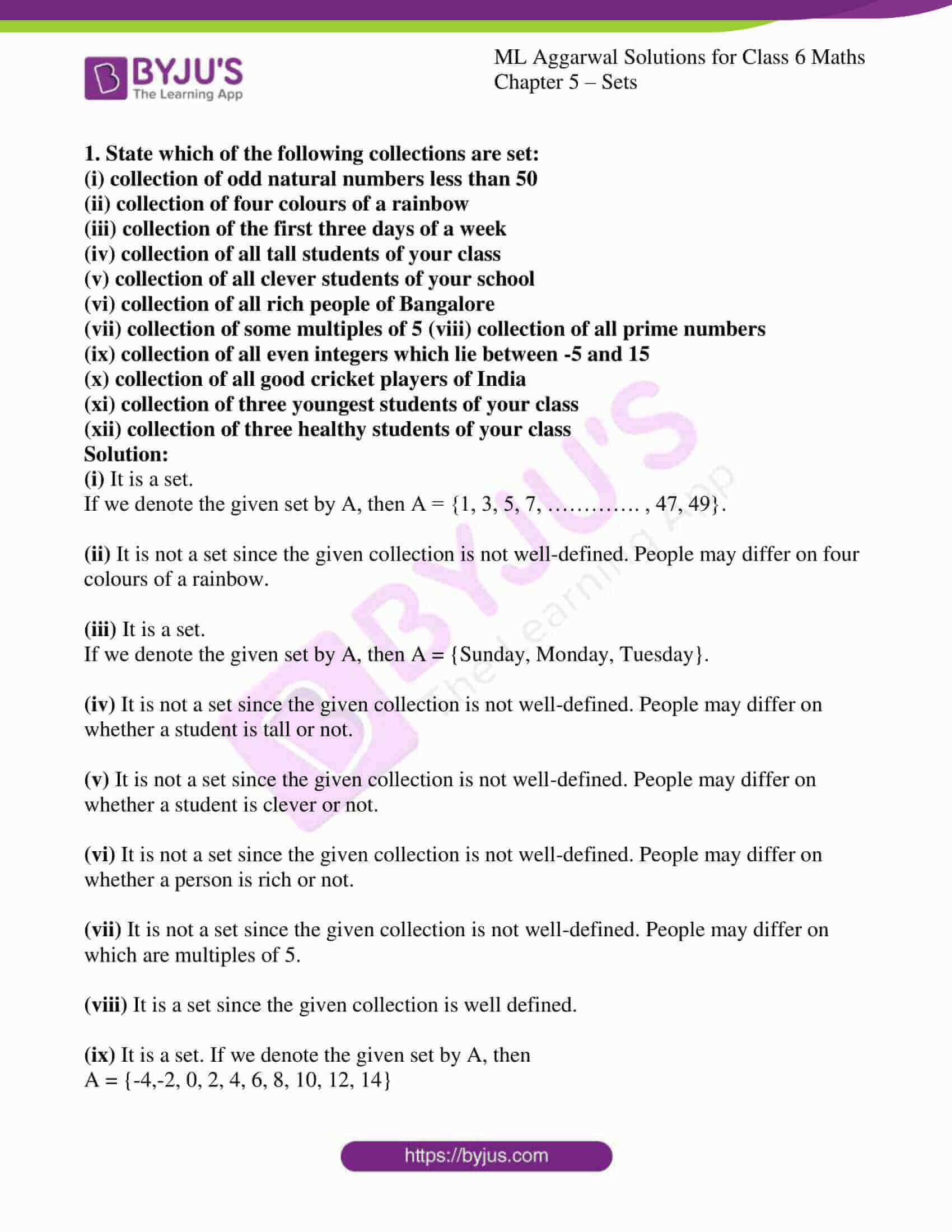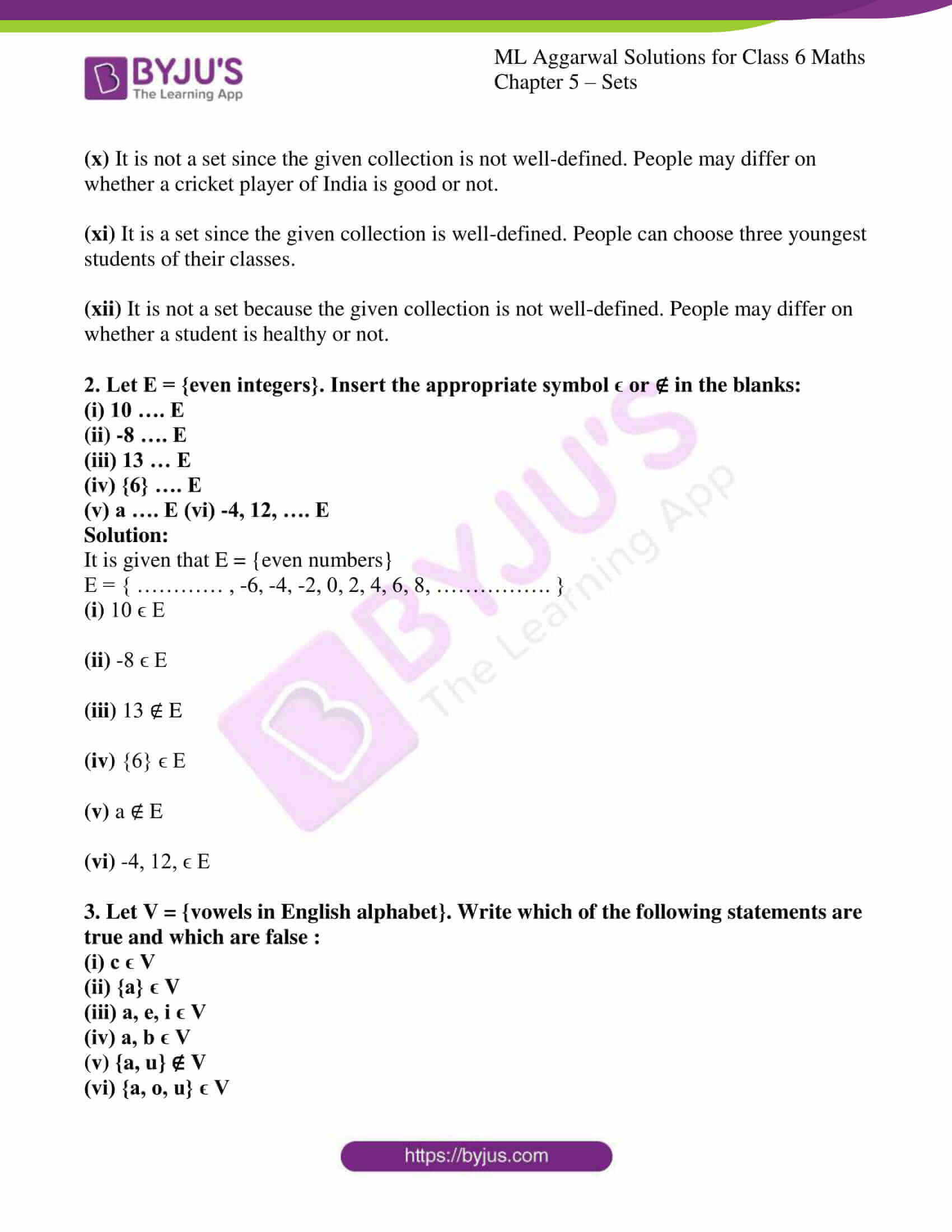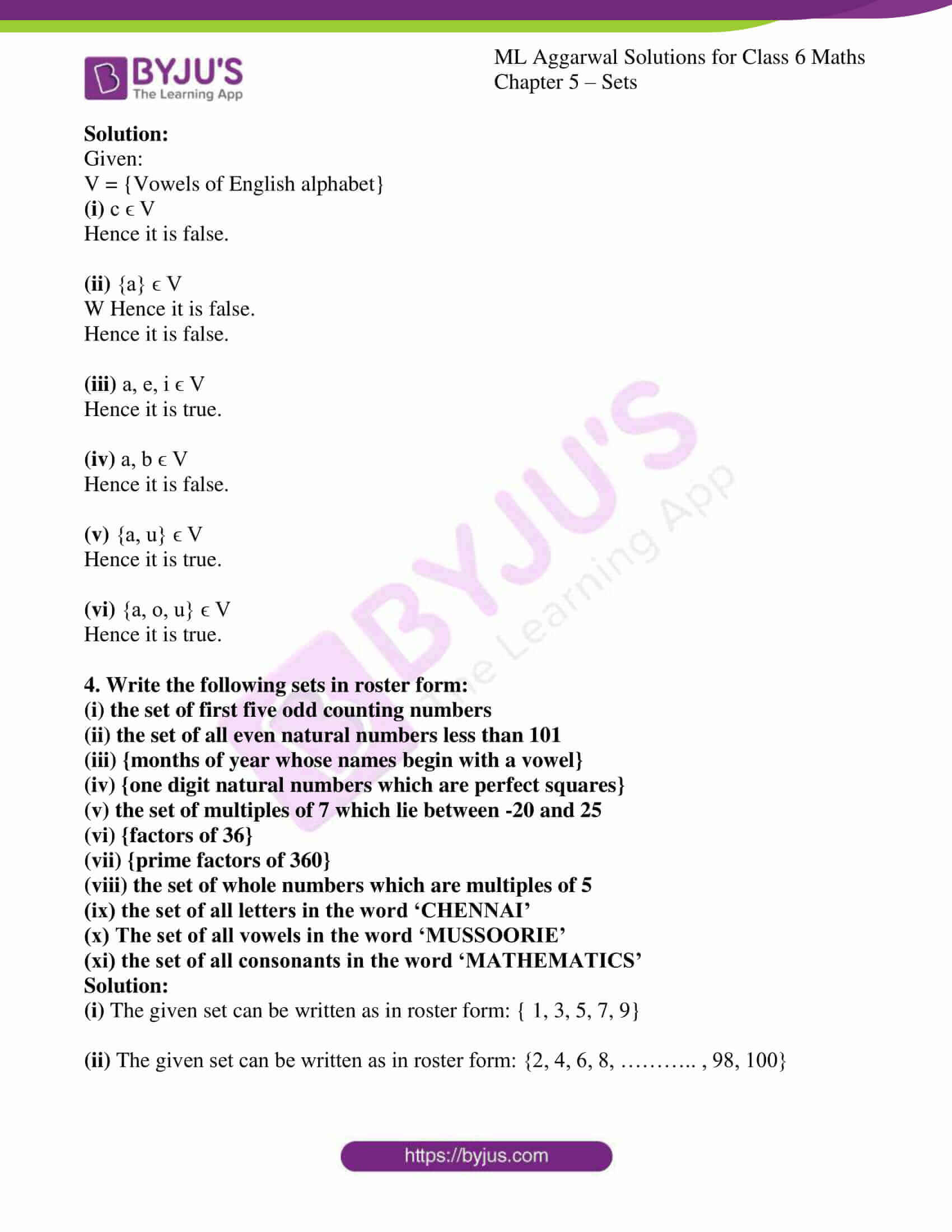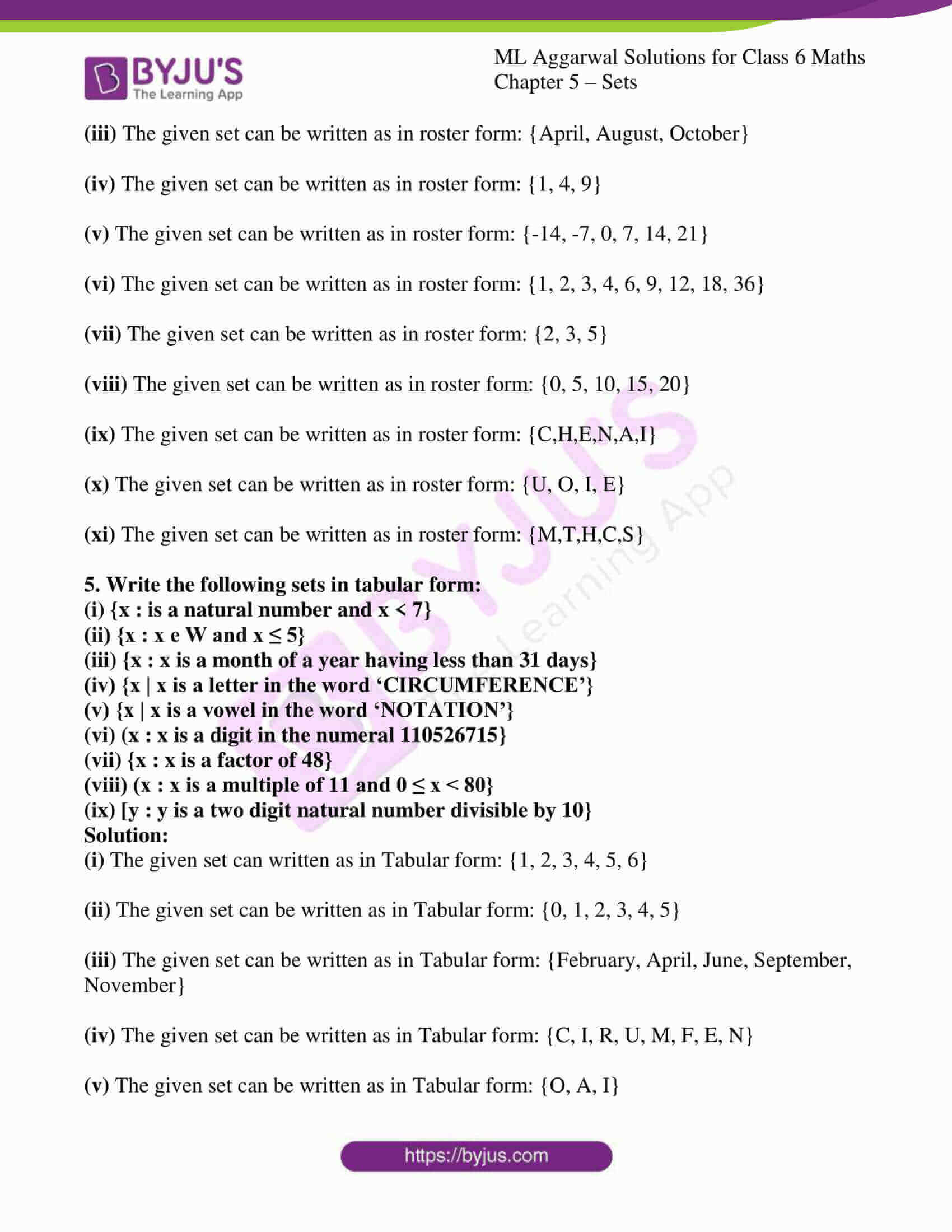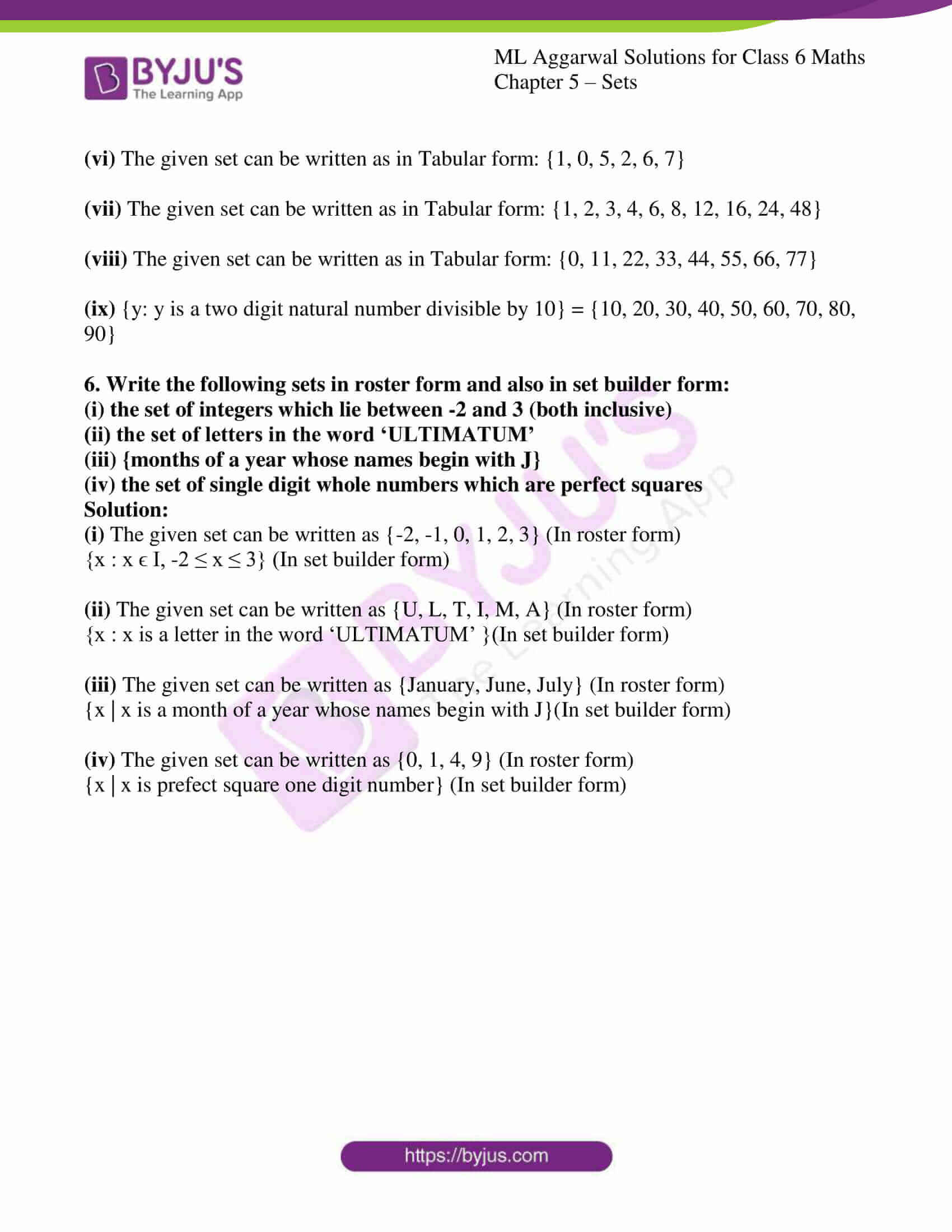### Access answers to ML Aggarwal Solutions for Class 6 Maths Chapter 5 – Sets

1. State which of the following collections are set:
(i) collection of odd natural numbers less than 50
(ii) collection of four colours of a rainbow
(iii) collection of the first three days of a week
(iv) collection of all tall students of your class
(v) collection of all clever students of your school
(vi) collection of all rich people of Bangalore
(vii) collection of some multiples of 5 (viii) collection of all prime numbers
(ix) collection of all even integers which lie between -5 and 15
(x) collection of all good cricket players of India
(xi) collection of three youngest students of your class
(xii) collection of three healthy students of your class
Solution:

(i) It is a set.

If we denote the given set by A, then A = {1, 3, 5, 7, …………. , 47, 49}.

(ii) It is not a set since the given collection is not well-defined. People may differ on four colours of a rainbow.

(iii) It is a set.

If we denote the given set by A, then A = {Sunday, Monday, Tuesday}.

(iv) It is not a set since the given collection is not well-defined. People may differ on whether a student is tall or not.

(v) It is not a set since the given collection is not well-defined. People may differ on whether a student is clever or not.

(vi) It is not a set since the given collection is not well-defined. People may differ on whether a person is rich or not.

(vii) It is not a set since the given collection is not well-defined. People may differ on which are multiples of 5.

(viii) It is a set since the given collection is well defined.

(ix) It is a set. If we denote the given set by A, then

A = {-4,-2, 0, 2, 4, 6, 8, 10, 12, 14}

(x) It is not a set since the given collection is not well-defined. People may differ on whether a cricket player of India is good or not.

(xi) It is a set since the given collection is well-defined. People can choose three youngest students of their classes.

(xii) It is not a set because the given collection is not well-defined. People may differ on whether a student is healthy or not.

2. Let E = {even integers}. Insert the appropriate symbol ϵ or ∉ in the blanks:
(i) 10 …. E
(ii) -8 …. E
(iii) 13 … E
(iv) {6} …. E
(v) a …. E (vi) -4, 12, …. E
Solution:

It is given that E = {even numbers}

E = { ………… , -6, -4, -2, 0, 2, 4, 6, 8, ……………. }

(i) 10 ϵ E

(ii) -8 ϵ E

(iii) 13 ∉ E

(iv) {6} ϵ E

(v) a ∉ E

(vi) -4, 12, ϵ E

3. Let V = {vowels in English alphabet}. Write which of the following statements are true and which are false :
(i) c ϵ V
(ii) {a} ϵ V
(iii) a, e, i ϵ V
(iv) a, b ϵ V
(v) {a, u} ∉ V
(vi) {a, o, u} ϵ V
Solution:

Given:

V = {Vowels of English alphabet}

(i) c ϵ V

Hence it is false.

(ii) {a} ϵ V

W Hence it is false.

Hence it is false.

(iii) a, e, i ϵ V

Hence it is true.

(iv) a, b ϵ V

Hence it is false.

(v) {a, u} ϵ V

Hence it is true.

(vi) {a, o, u} ϵ V

Hence it is true.

4. Write the following sets in roster form:
(i) the set of first five odd counting numbers
(ii) the set of all even natural numbers less than 101
(iii) {months of year whose names begin with a vowel}
(iv) {one digit natural numbers which are perfect squares}
(v) the set of multiples of 7 which lie between -20 and 25
(vi) {factors of 36}
(vii) {prime factors of 360}
(viii) the set of whole numbers which are multiples of 5
(ix) the set of all letters in the word ‘CHENNAI’
(x) The set of all vowels in the word ‘MUSSOORIE’
(xi) the set of all consonants in the word ‘MATHEMATICS’
Solution:

(i) The given set can be written as in roster form: { 1, 3, 5, 7, 9}

(ii) The given set can be written as in roster form: {2, 4, 6, 8, ……….. , 98, 100}

(iii) The given set can be written as in roster form: {April, August, October}

(iv) The given set can be written as in roster form: {1, 4, 9}

(v) The given set can be written as in roster form: {-14, -7, 0, 7, 14, 21}

(vi) The given set can be written as in roster form: {1, 2, 3, 4, 6, 9, 12, 18, 36}

(vii) The given set can be written as in roster form: {2, 3, 5}

(viii) The given set can be written as in roster form: {0, 5, 10, 15, 20}

(ix) The given set can be written as in roster form: {C,H,E,N,A,I}

(x) The given set can be written as in roster form: {U, O, I, E}

(xi) The given set can be written as in roster form: {M,T,H,C,S}

5. Write the following sets in tabular form:
(i) {x : is a natural number and x < 7}
(ii) {x : x e W and x ≤ 5}
(iii) {x : x is a month of a year having less than 31 days}
(iv) {x | x is a letter in the word ‘CIRCUMFERENCE’}
(v) {x | x is a vowel in the word ‘NOTATION’}
(vi) (x : x is a digit in the numeral 110526715}
(vii) {x : x is a factor of 48}
(viii) (x : x is a multiple of 11 and 0 ≤ x < 80}
(ix) [y : y is a two digit natural number divisible by 10}
Solution:

(i) The given set can written as in Tabular form: {1, 2, 3, 4, 5, 6}

(ii) The given set can be written as in Tabular form: {0, 1, 2, 3, 4, 5}

(iii) The given set can be written as in Tabular form: {February, April, June, September, November}

(iv) The given set can be written as in Tabular form: {C, I, R, U, M, F, E, N}

(v) The given set can be written as in Tabular form: {O, A, I}

(vi) The given set can be written as in Tabular form: {1, 0, 5, 2, 6, 7}

(vii) The given set can be written as in Tabular form: {1, 2, 3, 4, 6, 8, 12, 16, 24, 48}

(viii) The given set can be written as in Tabular form: {0, 11, 22, 33, 44, 55, 66, 77}

(ix) {y: y is a two digit natural number divisible by 10} = {10, 20, 30, 40, 50, 60, 70, 80, 90}

6. Write the following sets in roster form and also in set builder form:
(i) the set of integers which lie between -2 and 3 (both inclusive)
(ii) the set of letters in the word ‘ULTIMATUM’
(iii) {months of a year whose names begin with J}
(iv) the set of single digit whole numbers which are perfect squares
Solution:

(i) The given set can be written as {-2, -1, 0, 1, 2, 3} (In roster form)

{x : x ϵ I, -2 ≤ x ≤ 3} (In set builder form)

(ii) The given set can be written as {U, L, T, I, M, A} (In roster form)

{x : x is a letter in the word ‘ULTIMATUM’ }(In set builder form)

(iii) The given set can be written as {January, June, July} (In roster form)

{x | x is a month of a year whose names begin with J}(In set builder form)

(iv) The given set can be written as {0, 1, 4, 9} (In roster form)

{x | x is prefect square one digit number} (In set builder form)